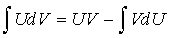Rectilinear Motion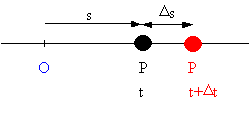Time: t

Position: s

Speed: v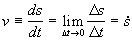Acceleration: a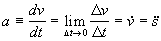Lemma: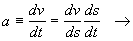Method for integration:

1.      Check to see if there are only two variables

2.      If you have only two variables, see if you can separate the variables (SV)

3.      If you have more than two variables or if your variables are not the ones you are looking for, use the lemma to see if you can work around the problem

4.      Once you have separated the variables, then integrate (I)

Acceleration as a function of time: a=f(t)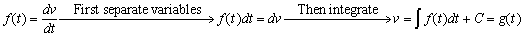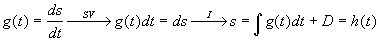C and D are constants of integration evaluated from initial conditions

Acceleration as a function of velocity: a=f(v)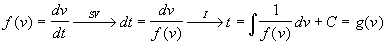If you can solve to get v=k(t), then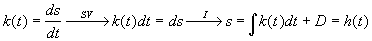or by using the lemma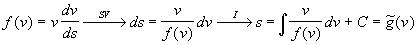Acceleration as a function of position: a=f(s)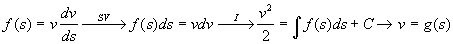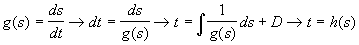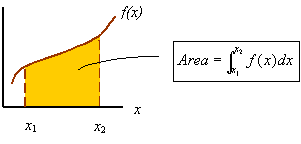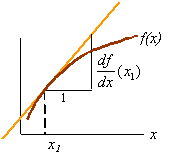Derivatives to know: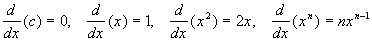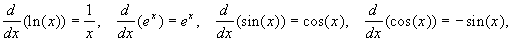The chain rule for derivatives: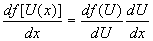Integrals to know: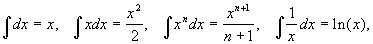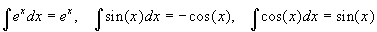Change of variables: Given a function U(x), one can use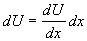to change the variable of integration from x to U for an integral of the form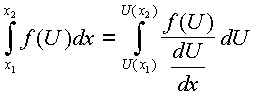Integration by parts: